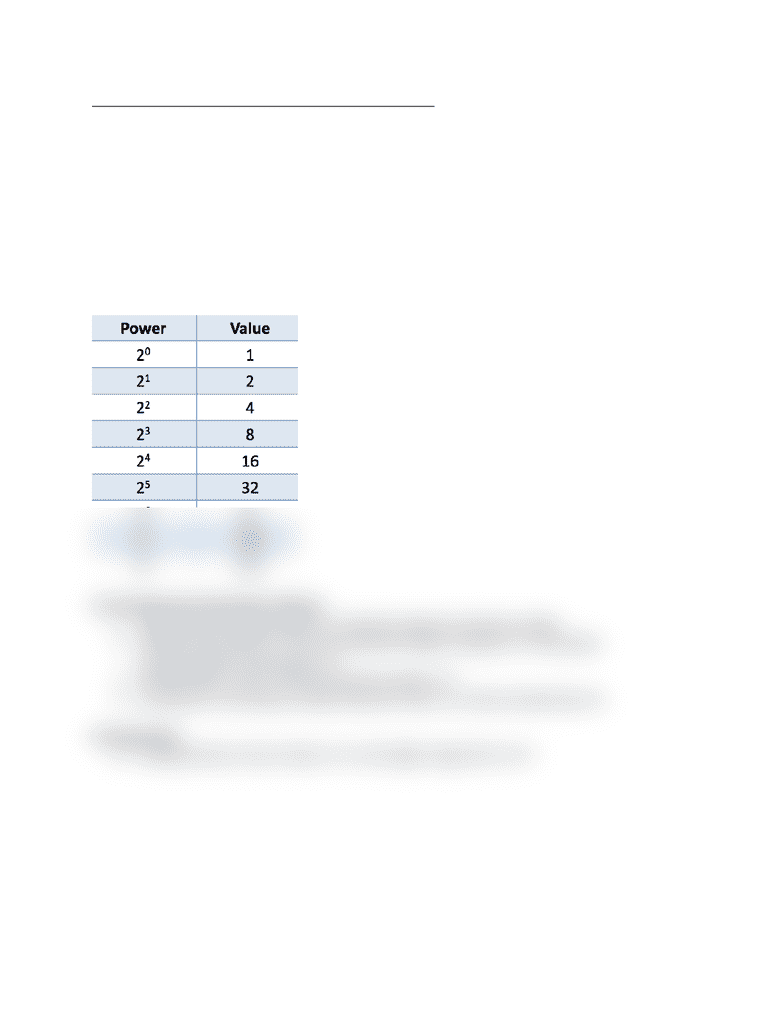# ITP 165x Lecture Notes - Lecture 24: Binary Number

38 views2 pagesITP 165: Intro to C++ - Lecture 24: Binary Refresher
Decimal Numbers
In decimal (base 10), every digit can range from 0 to 9
So 150 in decimal is equal to the value of 100 + 50 + 0 = 150 in decimal
Binary Numbers
Binary numbers are in base 2
This means that every digit can only be a 1 or a 0
So 101 in binary is 4 + 0 + 1 = 5 in decimal
Internally, computers use binary to represent all of their numbers
In order to easily convert from decimal to binary, you need to know the powers of 2
Converting from Decimal to Binary
1. Determine the largest power of 2 that fits inside the decimal number
2. Subtract that power of 2 from the decimal number, and put a 1 in the place
corresponding to that power of 2
3. Repeat steps 1 and 2 until the decimal number is 0
4. Any places in the binary number that don’t have 1s in them should have 0s
Hexadecimal (or hex) is base 16, so the digits range from 0 to F
Unlock document

This preview shows half of the first page of the document.
Unlock all 2 pages and 3 million more documents.

## Document Summary

Itp 165: intro to c++ - lecture 24: binary refresher. In decimal (base 10), every digit can range from 0 to 9. So 150 in decimal is equal to the value of 100 + 50 + 0 = 150 in decimal. This means that every digit can only be a 1 or a 0. So 101 in binary is 4 + 0 + 1 = 5 in decimal. Internally, computers use binary to represent all of their numbers. In order to easily convert from decimal to binary, you need to know the powers of 2. Hexadecimal (or hex) is base 16, so the digits range from 0 to f. The easy way is to break the binary number into groups of 4. So for example, 45 written in binary is 00101101. Treat each group of 4 bits as a separate 4-bit binary number, and convert each group of. Finally, convert each decimal number to the corresponding hex digit.

## Get access

\$10 USD/m
Billed \$120 USD annuallyHomework Help
Study Guides
Textbook Solutions
Class Notes
Textbook Notes
Booster Class